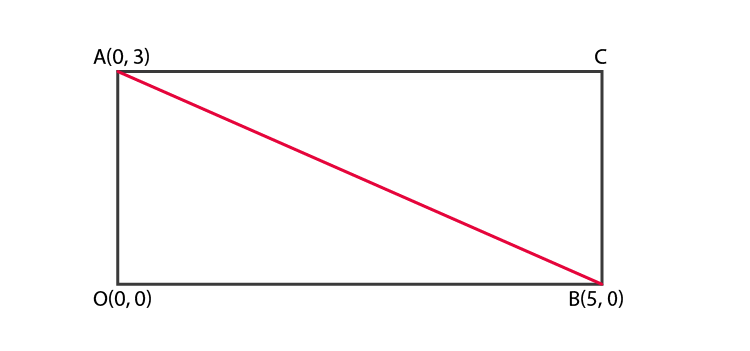# AOBC is a rectangle whose three vertices are vertices A (0, 3), O (0, 0) and B (5, 0). The length of its diagonal is (A) 5 (B) 3 (C) √34 (D) 4

The correct answer is (C) √34

The three vertices are: A = (0, 3), O = (0, 0), B = (5, 0)

The diagonals of a rectangle are of equal length,

Length of the diagonal AB = Distance between the points A and B

We know that the Distance formula: d2 = (x2 – x1)2 + (y2 – y1)2As per the given details

x1 = 0, x2 = 5

y2 = 3, y2 = 0

d2 = (5 – 0)2 + (0 – 3)2

d= √((5-0)2+ (0-3)2)

d = √(25 + 9)= √34

Distance between A (0, 3) and B (5, 0) is √34

Therefore, the length of its diagonal is √34(0)(1)